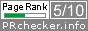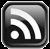# LEARN MS-ACCESS TIPS AND TRICKS

Learn Microsoft Access Advanced Programming Techniques, Tips and Tricks.

### Introduction

Last week we have explored the usage of ByVal (By Value) and ByRef (By Reference),  in the Function Parameter, to pass the value from a Variable from the calling function or the Location Address of a Variable to the Called Function.  If you have not visited the earlier page the link is given below:

Now, we will learn:

1. how to pass the location address of a single element of an Array  to the called Function and change its value
2. how to pass the Location Address of an array and sort the values in the array in Descending Order.

### Two Test Programs.

#### Function ArrayArg_Test1().

First, let us write two small programs for the first example. The first Program Code is given below.

```Public Function ArrayArg_Test1()
Dim NumArray(1 To 5) As Integer, j As Integer

'load array with numbers 1 to 5
For j = 1 To 5
NumArray(j) = j
Next

'pass a single element value
Call ArrayArg_Test2(NumArray(4))

'Print the Array values after change
For j = 1 To 5
Debug.Print j, NumArray(j)
Next

End Function

```
```'Result:
1
2
3
20
5
```

In the first line of code, we have defined an Array Variable (NumArray()) with five elements to store Integer type values. Variable j is a control variable defined for For...Next Loop.

Inside the For....Next loop the Array is loaded with values 1 to 5, i.e. NumArray(1) = 1, Numarray(2)=2 and so on up to 5 elements.

Next, we call the function ArrayArg_Test2() function with the NumArray(4) element passed as parameter.  The number 4 within brackets is the index number of the element, not the value itself.  But, we have the value 4 in that element too.  The called function ArrayArg_Test2() receives the passed value/location depends on the parameter definition there. If we use ByRef or omit the ByRef specification before the Variable Name and Data Type then the called function takes the passed variable's location address to work with the value stored in the original Variable.  We will go with the second method and will not use the ByRef specification in the next function’s parameter definition.

Within the next For...Next loop the array contents are printed in the Debug Window.  If any change done by the function ArrayArg_Test2() will show up in the printed list.  Since we already knew that the array elements 1 to 5 contain the values 1,2,3,4,5 we have not printed those values before calling the second function.

#### Function ArrayArg_Test2().

The ArrayArg_Test2() Function VBA Code is given below:

```Public Function ArrayArg_Test2(NA As Integer) 'The word ByRef is omited
'multiply NumArray(4) value * 5 = 20
NA = NA * 5
End Function
```

The Variable NA is assigned with the NumArray’s 4th element location address.  The ArrayArg_Test2() picks the value from NumArray(4) itself, multiply it by 5 and store the result back into the same location.

This was working with a single element of an Array.  What about passing the full Array’s location address and work with hundreds of elements of this array in the called Function.

### Get the Array Sorted and Print.

We will pass the same array, we have used in the above example, as the parameter, to sort the values in descending order with the Sorting function

The modified version of the First Function Code is given below.

```Public Function ArrayArg_Test3()
Dim NumArray(1 To 5) As Integer, j As Integer

For j = 1 To 5
NumArray(j) = j
Next

'Pass the array to the called function
Call ArrayArg_Test4(NumArray())

'Print the Sorted Array
For j = 1 To 5
Debug.Print j, NumArray(j)
Next

End Function
```

Check the function call statement. NumArray() is passed without the element number, as we did in the earlier example. The opening and closing brackets are required along with the array variable name to indicate that the parameter passed is an array not a single variable.

When control is returned from the ArrayArg_Test4() function the sorted list of numbers is printed in the debug window. The value printed at the left side is the array element number and the right-side value is the array value itself,  sorted in descending order.

#### Sort the Array in Descending (Z-A) Order.

The Data Sorting Program is given below:

```Public Function ArrayArg_Test4(apple() As Integer)
Dim j As Integer, k As Integer, Temp As Integer

'Bubble Sort the Array in Descending order
' 1st loop runs maximum array elements minus 1 times

For j = 1 To 5 - 1 ' in place of 5-1 you may use Ubound(apple)-1

' inner loop starts with outer loop's current value + 1
' and runs to the maximum number of array elements times

For k = j + 1 To 5 ' replace 5 with Ubound(apple)

If apple(k) > apple(j) Then 'if second value is greater

Temp = apple(j) 'copy 1st value to Temp Variable

apple(j) = apple(k) 'move greater value up

apple(k) = Temp ' move the smaller value down

End If
Next k ' compare next two elements
Next j
End Function

```

To Sort the values in Ascending Order then the only change in this program required is to change Greater Than logic (>) symbol to the Less Than (<) symbol. The number we have loaded into the array was already in Ascending Order.

If you remove the 5-1 constant from the first For...Next loop and replace with Ubound(apple)-1 and replace 5 with Ubound(apple) in the second loop you can use the program to sort the array with any number of elements without a change in the Program.

Notice that we have omitted the ByRef specification in the called ArrayArg_Test4()Function Parameter definition. VBA, by default, takes it as ByRef Parameter Variable.

Share:

Comments subject to moderation before publishing.

## TranslateSubscribe in a readerYour email address:

Delivered by FeedBurner